# Hilbert polynomial

(diff) ← Older revision | Latest revision (diff) | Newer revision → (diff)

of a graded module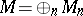A polynomial expressing the dimensions of the homogeneous components of the module as a function offor large natural numbers. More exactly, the following theorem, demonstrated in essence by D. Hilbert, is valid. Letbe a ring of polynomials over a field, graded so that theare homogeneous elements of the first degree, and letbe a graded-module of finite type; then there exists a polynomial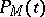with rational coefficients such that, for sufficiently large,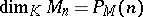. This polynomial is called the Hilbert polynomial.

Of greatest interest is the interpretation of the Hilbert polynomial of a graded ringwhich is the quotient ring of the ringby a homogeneous ideal; in such a case the Hilbert polynomial gives the projective invariants of the projective variety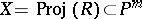defined by the ideal. In particular, the degree of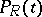coincides with the dimension of, whileis said to be the arithmetic genus of. Hilbert polynomials also serve to express the degree of the imbedding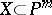. The Hilbert polynomial of the ringis also the name given to the Hilbert polynomial of the projective varietywith respect to the imbedding. Ifis the invertible sheaf corresponding to this imbedding, then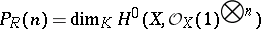for sufficiently large.

How to Cite This Entry:
Hilbert polynomial. Encyclopedia of Mathematics. URL: http://encyclopediaofmath.org/index.php?title=Hilbert_polynomial&oldid=14449
This article was adapted from an original article by V.I. Danilov (originator), which appeared in Encyclopedia of Mathematics - ISBN 1402006098. See original article